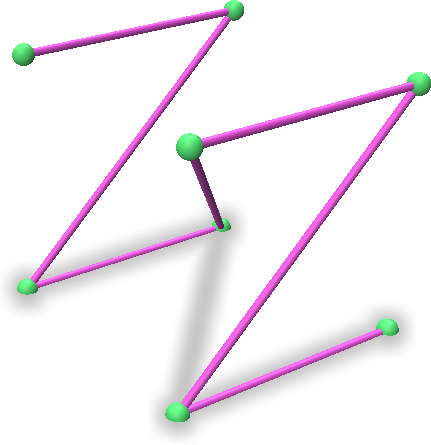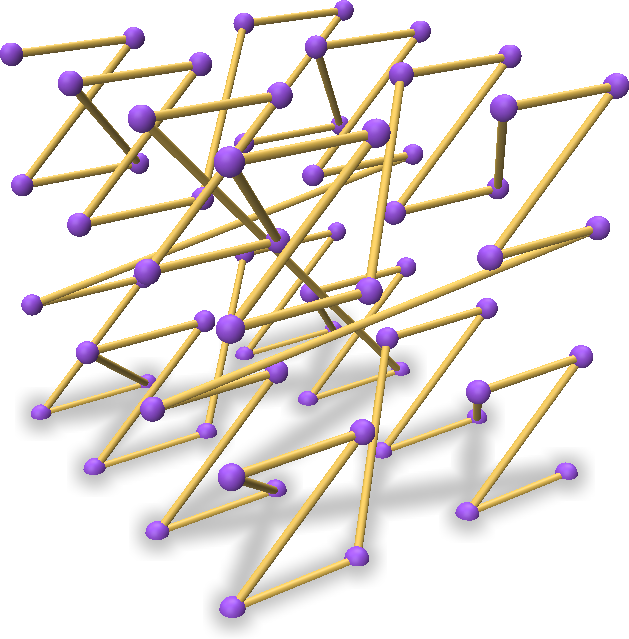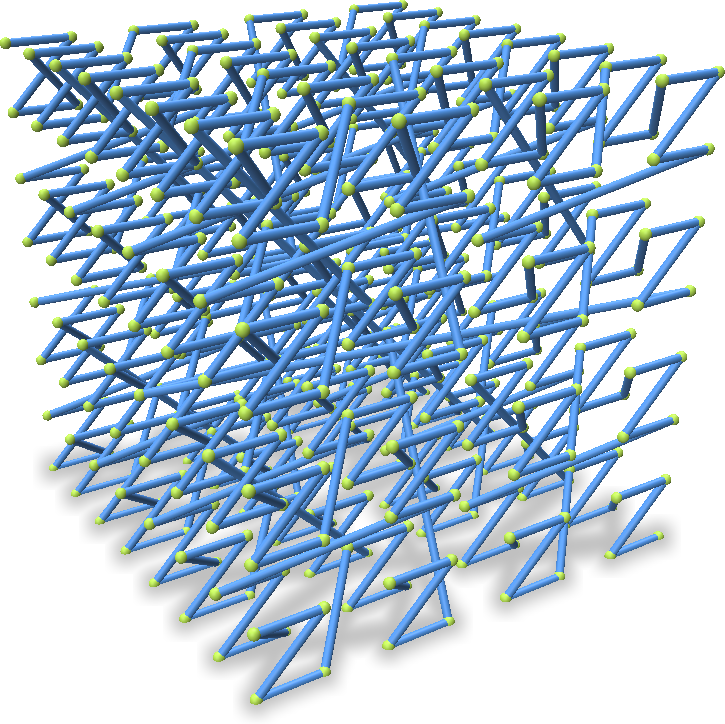# Morton Encoding/Decoding a Point Cloud

Point Cloud Utils has a number of tools for operating on Morton Encoded point clouds. Morton encoding works by projecting points onto a space filling Z-Curve (illustrated below), and recording the distance along this curve from the origin.Morton Encoding point clouds has a number of useful application, such as approximate-k-nearest neighbor search, locality-sensitive point hashing, and point sorting, to name a few.

To morton encode a point cloud, you must first convert the point coordinates to integers via quantization. The Point Cloud Utils morton coding utility uses 64 bits and can thus represent points in $$x \in [-1048576, 1048576]^3 \subset \mathbb{Z}^3$$.

## Morton Encoding and Decoding Points

You can quickly encode/decode points to/from Morton codes by quantizing them to integer coordinates and calling morton_encode/morton_decode.

import numpy as np
import point_cloud_utils as pcu

# quantize points to bins of size 1/128
# NOTE: quantized point coordinates must lie between -1048576 and 1048576
eps = 1.0 / 128.0
pts_quantized = (pts / eps).astype(np.int32)

# Convert points to morton codes
# morton_codes has shape [pts.shape,] (one code per point)
morton_codes = pcu.morton_encode(pts_quantized)

# Decode morton codes back to integer coordinates
pts_decoded = pcu.morton_decode(morton_codes)


## Approximate K-Nearest-Neighbor Search with Morton Coding

Finding the nearest points in Morton space to a set of query points is a very fast aproximate KNN algorithm. This can be done via morton_knn:

import numpy as np
import point_cloud_utils as pcu

# Sample random KNN query points in a bounding box 1.1x larger than the object
query_pts = np.random.rand(100, 3) * (pts.max(0) - pts.min(0)) * 1.1 - pts.min(0)

# quantize points to bins of size 1/128
# NOTE: quantized point coordinates must lie between -1048576 and 1048576
eps = 1.0 / 128.0
pts_quantized = (pts / eps).astype(np.int32)
query_pts_quantized = (query_pts / eps).astype(np.int32)

# Convert points and query points to morton codes
# morton_codes has shape [pts.shape,] (one code per point)
morton_codes = pcu.morton_encode(pts_quantized)
query_codes = pcu.morton_encode(query_pts_quantized)

# Number of neighbors per point
num_nbrs = 7

# knn_idx is an array of size [query_pts.shape, num_nbrs]
# where knn_idx is a vector of indices into pts/morton_codes
# of the approximate k nearest neighbors (sorted)
knn_idx = pcu.morton_knn(morton_codes, query_codes, num_nbrs)


## Sorting Points Along a Morton Curve

Morton codes can be used to impose a sort order on 3D points by first quantizing them and the quantized points in Morton order.

import numpy as np
import point_cloud_utils as pcu

# quantize points to bins of size 1/128
# NOTE: quantized point coordinates must lie between -1048576 and 1048576
eps = 1.0 / 128.0
pts_quantized = (pts / eps).astype(np.int32)

# Convert points and query points to morton codes
# morton_codes has shape [pts.shape,] (one code per point)
morton_codes = pcu.morton_encode(pts_quantized)

# Permute the points to sort them by their morton code
sorted_pts = pts[np.argsort(morton_codes)]


## Adding and Subtracting Coordinates in Morton Space

You can add and subtract Morton encoding of points directly via morton_add and morton_subtract

import numpy as np
import point_cloud_utils as pcu

# Let's generate some random noise and add it to the morton
# encoded points
offsets = np.random.randn(*pts.shape) * 0.02

# quantize points and offsets to bins of size 1/128
# NOTE: quantized point coordinates must lie between -1048576 and 1048576
eps = 1.0 / 128.0
pts_quantized = (pts / eps).astype(np.int32)
offsets_quantized = (offsets / eps).astype(np.int32)

# Convert points and query points to morton codes
# morton_codes has shape [pts.shape,] (one code per point)
morton_codes = pcu.morton_encode(pts_quantized)

# Convert offset to morton codes
offset_codes = pcu.morton_encode(offsets_quantized)

# Add noise offsets in Morton space